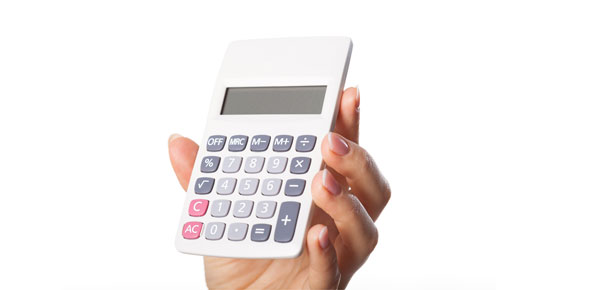# Intermediate 2 Percentages

5 QuestionsSettingsThis quiz will test you on percentages. You may need a calculator.

Related Topics
• 1.
What is ment by the tem appreciation?
• A.

The decrease in value of something.

• B.

The increase in value of something.

• C.

The rate of change.

• 2.
1.41 is what as a percentage?
• A.

41%

• B.

141%

• C.

Decimals can not be converted to percentages.

• 3.
The Population of a town goes up by 12% each year. The population is 14000. Caluculate the population in 5 years. Give your answer to the nearest thousand.
• 4.
A car  of value £8000 goes down in value 10 percent per year for four years. Calculate the cars new value in four years. Give your answer to the nearest pound.
• 5.
InterCalculate the compound interest gained on £500 invested for ten years at 4% interest. Give your answer to the nearest pound.